# SAT Part 3 — Reducing ILP to SAT

This is the third part of the SAT series. For your convenience you can find other parts in the table of contents in Part 1 — Boolean logic

Today we are going to reduce ILP to SAT.

# Introduction

Depending on the solver, typical ILP supports the following operations:

2. Negating variable
3. Subtracting variables
4. Multiplying variable by constant
5. Dividing variable by constant
7. Adding greater or equal constraint
8. Adding less or equal constraint
10. Marking variable as integer

Some operations are obviously represented in SAT while others cannot be easily reduced.

If we assume that integers are of a specific width – which is not a crazy assumption as we use computers anyway and they do use fixed width numbers for increased performance – then we can implement operations 1-3 easily.

Operation 4 can be easily implemented as well assuming we work with integers only.

Operation 5 is tricky as the result may not be an integer so we can’t represent it properly.

Operation 6 is straightforward. So are operations 7 and 8 when we use slack variables.

Operation 9 is something we cannot implement in pure SAT. There is MAXSAT where we can optimize for a goal function, though.

Operation 10 is obvious.

# Reduction

First we reduce the ILP problem to its canonical form. We replace all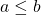constraints with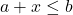where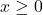. Same for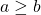– we change it to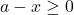. We can stay with negative variables or replace them with subtraction and a slack variable.

Next, we reduce the canonical form to 0-1 ILP. We take every integer and replace it with bits.

Then, we remove the goal function. Ultimately we end up with a matrix of equalities of integer variables.

Now, we implement operations 1-4 and 6 using the approach from previous parts of this series.

# Analysis

How slow is this going to be? For each integer we effectively introduce 32 clauses. For multiplication we need to add additional 32 partial sums which we then add together to get the result.

So, when we reduce the ILP model multiplying two integers with 27 bits width we end up with 2260029 clauses and 8409792 conjunctions. The model can be then solved in less than 2 minutes on my personal laptop. The DIMACS model has 210 MBs. For exponentiation it’s even bigger – 25375974 variables, 94454003 conjunctions, and 2.5 GBs.

Similarly, Nondeterministic Turing Machine for palindromes (the one we implemented in ILP) has 22123252 variables, 79175097 conjunctions, and 2.1 GBs.

That’s big. But it works and now we can use plenty of SAT solvers to calculate ILP models.